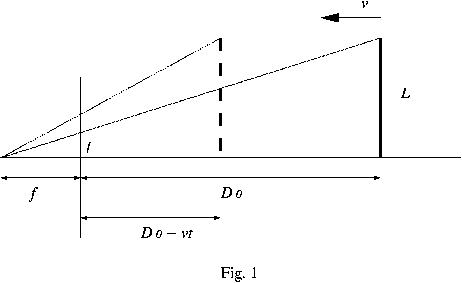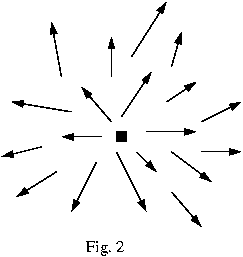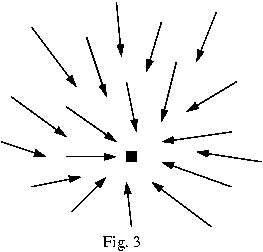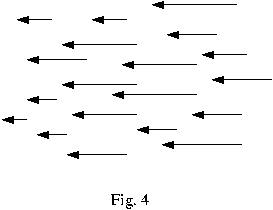Motion Analysis

Course Notes by Malvika Rao

Presented to Prof. Kaleem Siddiqi for CS558,
School of Computer Science, McGill University.

Introduction

The study of motion in computer vision enables us to extract visual information from the spatial and temporal changes occurring in an image sequence. An image sequence is defined to be a series of n images, or frames, acquired at discrete times intervals tk = t0 + k * dt, where dt is a fixed time interval, and k = 0, 1,..., n - 1. Assuming unvarying illumination conditions, changes in an image sequence are caused by a relative motion between the camera and the scene: the viewing camera could move in front of a static scene, or parts of the scene could move in front of a stationary camera, or, in general, both camera and objects could be moving with different motions. Visual motion is important for 2 main reasons. First, the apparent motion of objects onto the image plane is a strong visual cue for understanding structure and 3D motion. Second, biological visual systems use visual motion to infer properties of the 3D world with little a priori knowledge of it. The following simple examples illustrate these points:

Example 1: Random Dot Sequences

Consider an image of random dots, generated by assigning to each pixel a random grey level. Consider a second image obtained by shifting a squared, central region of the first image by a few pixels, say, to the right, and filling the gap thus created with more random dots. Displaying the 2 images in sequence, one after the other at a sufficiently fast rate, will induce the viewer to see a square moving sideways back and forth against a steady background. When the 2 images are, instead, viewed one placed next to the other, such that each eye can see only one image, the impression of a square floating against the background is produced. The latter case can be attributed to depth perception due to the disparity between parts of the 2 images.

Example 2: Computing Time-to-Collision

Consider a planar version of the pinhole camera model, and a vertical bar perpendicular to the optical axis, travelling towards the camera with constant velocity as shown in Fig. 1. It is possible to compute the time, T, taken by the bar to reach the camera only from image information, without knowing either the real size of the bar or its velocity in 3D space.Let L denote the real size of the bar, V its constant velocity, and f the focal length of the camera. The origin of the reference frame is the projection centre. If the position of the bar on the optical axis is D(0) at time t = 0, its position at a later time t is given by D = D(0) - V * t. From Fig. 1 we see that the apparent size of the bar at time t on the image plane is given by

l(t) = f * L / D.

If we compute the time derivative of l(t) we get

l'(t) = (f * L * V) / D2.

Taking the ratio l(t) / l'(t), we obtain,

T = l(t) / l'(t).

Substituting the expressions for l(t) and l'(t) we get the time to collision to be

T = D / V.

The Problem of Motion Analysis

The motion analysis problem can be divided into 2 subproblems: that of correspondence, and that of reconstruction. The correspondence problem states: which elements of a frame correspond to which elements of the next frame of the sequence? The reconstruction problem states: given a number of corresponding elements, and possibly knowledge of the camera's intrinsic parameters, what can be said about the 3D motion and structure of the observed world? These problems are often solved using optical flow. Before we proceed to discuss more of the motion analysis problem we make the following simplifying assumption:

Assumption

There is only one, rigid, relative motion between the camera and the observed scene, and the illumination conditions do not vary.

The above stated assumption implies that the 3D objects observed cannot move with different motions. This assumption is violated, for example, by sequences of football matches, motorway traffic, or busy streets, but is satisfied by, say, the sequence of a building viewed by a moving observer. This assumption also excludes flexible objects, and nonrigid motions such as stretching and shearing; for example, deformable objects such as clothes moving are ruled out.

The Motion Field of Rigid Objects

The motion field is the 2D vector field of velocities of the image points, induced by the relative motion between the viewing camera and the observed scene. The motion field can be thought of as the projection of the 3D velocity field on the image plane.

Let P = [X, Y, Z]T be a 3D point in the camera reference frame, where the centre of projection is the origin, the optical axis is the Z axis, and f denotes the focal length. The image of a scene point, P, is the point, p, given by

p = f * P / Z.

Since the third coordinate of p is always equal to f, we write p = [x, y]T instead of p = [x, y, f]T. The velocity of the 3D point P can be described as

V = -T - w x P,

where T is the translational component of the motion, and w the angular velocity. Breaking the 3D velocity, V, into its components yields:

Vx = -Tx - (wy * Z) + (wz * Y)

Vy = -Ty - (wz * X) + (wx * Z)

Vz = -Tz - (wx * Y) + (wy * X).

Taking the time derivative on both sides of equation 1, we obtain the 2D velocity of point p:

v = f * (Z * V - Vz * P) / Z2.

Since the component of the motion field along the optical axis is always equal to 0, we write v = [vx, vy]T instead of v = [vx, vy, 0]T. Substituting for V, Vz, and P in the equation for the 2D velocity, v, and breaking v into its components yields:

vx = ((Tz * x - Tx * f) / Z) - (wy * f) + (wz * y) + (wx * x * y / f) - (wy * x2 / f)

vy = ((Tz * y - Ty * f) / Z) + (wx * f) - (wz * x) - (wy * x * y / f) + (wx * y2 / f).

Notice that the motion field is the sum of 2 components, one of which depends on translation only, the other on rotation only. In particular, the translation components of the motion are

vxT = ((Tz * x - Tx * f) / Z)

vyT = ((Tz * y - Ty * f) / Z),

and the rotational components are

vxw = - (wy * f) + (wz * y) + (wx * x * y / f) - (wy * x2 / f)

vyw = (wx * f) - (wz * x) - (wy * x * y / f) + (wx * y2 / f).

NOTE: Only vxT and vyT depend on the depth, Z. The part of the motion field that depends on angular velocity does not carry information on depth.

Special Case 1: Pure Translation

This is the case in which the relative motion between the viewing camera and the scene has no rotational component. Since w = 0, the 2D velocity components reduce to

vx = (Tz * x - Tx * f) / Z

vy = (Tz * y - Ty * f) / Z.

Case Tz does not equal 0: We first consider the case where Tz does not equal 0. Let us introduce a point p0 = [x0, y0]T such that

x0 = f * Tx) / Tz

y0 = f * Ty) / Tz.

The 2D velocity components can now be written as

vx = (x - x0) * Tz / Z

vy = (y - y0) * Tz / Z.

The 2 equations obtained above indicate that the motion field of a pure translation is radial. In other words, the motion field is composed of vectors radiating from a common origin, the point p0, which is termed the vanishing point of the translation direction. Specifically, if Tz < 0, the vectors point away from p0, and p0 is called the focus of expansion (Fig. 2). If, on the other hand, Tz > 0, the vectors of the motion field point towards p0, and p0 is called the focus of contraction (Fig. 3). As well, the magnitude of these motion field vectors is directly proportional to the distance between p and p0, and inversely proportional to the depth, Z, of the 3D point P.Case Tz = 0: In this case the 2D velocity components become

vx = -f * Tx / Z

vy = -f * Ty / Z.

Notice that the numerators in the above 2 equations, namely, Tx, Ty, and f are constant everywhere in the 3D scene. Therefore, all the motion field vectors are parallel and their magnitudes are inversely proportional to the depth of the corresponding points (Fig. 4).Motion Parallax

Motion parallax occurs when 2 3D points project to the same 2D location. Let P, and P' be 2 different 3D points with projections p, and p'. The motion field for p, v consists of its components

vx = vxT + vxw

vy = vyT + vyw.

Likewise, the motion field v' of the 2D point p' can be expressed as

v'x = v'xT + v'xw

v'y = v'yT + v'yw.

If, at some time instant t, the 2D points p, and p' are coincident, we have p = p' = [x, y]T. Consequently, vxw = v'xw and vyw = v'yw. We can now define the relative motion field as the vector (dvx, dvy) where

dvx = vxT - v'xT = (Tz * x - Tx * f) * (1 / Z - 1 / Z')

dvy = vyT - v'yT = (Tz * y - Ty * f) * (1 / Z - 1 / Z').

Notice that the relative motion field does not depend on the rotational component of motion. Other factors being equal, dvx and dvy increase with the separation in depth between P and P'. The ratio between dvx and dvy can also be written as

dvx / dvy = (y - y0) / (x - x0),

where [x0, y0]T are the coordinates of the vanishing point.